## ML Aggarwal Class 8 Solutions for ICSE Maths Chapter 9 Direct and Inverse Variation Ex 9.3

Question 1.
A farmer can reap a field in 10 days while his wife can do it in 8 days (she does not waste time in smoking). If they work together, in how much time can they reap the field ?
Solution:
∵ Farmer can reap a field in 10 days
∴ In 1 day farmer can reap field = $$\frac{1}{10}$$
∵ farmer’s wife can reap a field in 8 days
∴ In 1 day farmer’s wife can reap field = $$\frac{1}{8}$$
∴ Farmer and his wife can reap field in 1 day $$\frac{1}{10}+\frac{1}{8}=\frac{4+5}{40}=\frac{9}{40}$$
∴ Farmer and his wife reap field in = $$\frac{40}{9}$$ days i.e. $$4 \frac{4}{9}$$ days.

Question 2.
A can do $$\frac{1}{5}$$th of a certain work in 2 days and B can do $$\frac{2}{3}$$rd of it in 8 days. In how
much time can they together complete the work ?
Solution:
A can do $$\frac{1}{5}$$th of a certain work in = 2 days
B can do $$\frac{2}{3}$$rd of a certain work in = 8 days
A’s, 1 day work = $$\frac{1}{5} \times \frac{1}{2}=\frac{1}{10}$$
B’s, 1 day work = $$\frac{2}{3} \times \frac{1}{8}=\frac{1}{12}$$
(A + B)’s 1 day work = $$\frac{1}{10}+\frac{1}{12}$$Question 3.
One tap fills a tank in 20 minutes and another tap fills it in 12 minutes. The tank being empty and if both taps are opened together, in how many minutes the tank will be full ?
Solution:
∵ First tap fill a tank in 20 minutes
∴ Second tap fill a tank in 12 minutes
∴ In 1 minutes first tank fill = $$\frac{1}{20}$$ parts
∴ In 1 minutes 2nd tank fill = $$\frac{1}{12}$$ parts
∴ In 1 minutes 1st and 2nd tank fill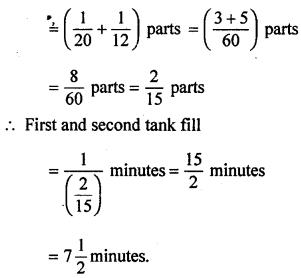Question 4.
A can do a work in 6 days and B can do it in 8 days. They worked together for 2 days and then B left the work. How many days will A require to finish the work?
Solution:
A can do a work in = 6 days
B can do a work in = 8 days
∴ A’s, 1 day work = $$\frac{1}{6}$$Question 5.
A can do a piece of work in 40 days. He works at it for 8 days and then B finishes the remaining work in 16 days. How long will they take to complete the work if they do it together?
Solution:
A can do a piece of work in = 40 days
∴ A’s, 1 day work = $$\frac{1}{40}$$
∴ A’s, 8 day work = $$\frac{8}{40}=\frac{1}{5}$$
Remaining work = $$1-\frac{1}{5}$$ = $$\frac{5-1}{5}=\frac{4}{5}$$
∵ B can do $$\frac{4}{5}$$ piece of work in = 16 daysQuestion 6.
A and B separately do a work in 10 and 15 Solution: days respectively. They worked together for some days and then A completed the remaining work in 5 days. For how many days had A and B worked together?
Solution:
A can do a work in = 10 days
B can do a work in = 15 days
A’s, 1 day work = $$\frac{1}{10}$$ …….(i)
B’s, 1 day work = $$\frac{1}{15}$$
(A + B)’s 1 day work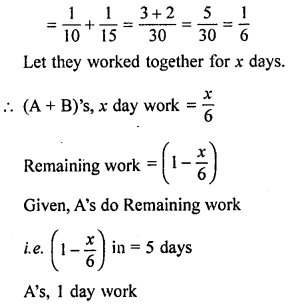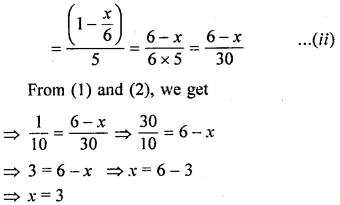Hence A and B can do work together in 3 days.

Question 7.
If 3 women or 5 girls take 17 days to complete a piece of work, how long will 7 women and 11 girls working together take to complete the work?
Solution:
Since 3 women’s work = 5 girl’s work
∴ 1 women’s work = $$\frac{5}{3}$$ girl’s work
∴ 7 women’s work = $$\frac{5}{3}$$ × 7 girl’s work = $$\frac{35}{3}$$ girls’ s work
∴ 7 women and 11 girls works = $$\frac{35}{3}$$ + 11 girl’s work = $$\frac{68}{3}$$ girl’s work
Since 5 girls can do the work in 17 days
∴ 1 girl can do the work in 17 × 5 days
∴ $$\frac{68}{3}$$ girls can do the work in $$\frac{17 \times 5}{\left(\frac{68}{3}\right)}$$ days
= $$\frac{17 \times 5 \times 3}{68}$$ days
= $$\frac{15}{4}$$ days = $$3 \frac{3}{4}$$ days
Hence 7 women and 11 girls can do the complete work in $$3 \frac{3}{4}$$ days.

Question 8.
A can do a job in 10 days while B can do it in 15 days. If they work together and earn ₹3500, how should they share the money ?
Solution:
A can do a job in 10 days
Then in one day A can do job = $$\frac{1}{10}$$
B can do a job in 15 days
Then in one day B can do job = $$\frac{1}{15}$$
Both A and B can do job in one day = $$\frac{1}{10}+\frac{1}{15}=\frac{3+2}{30}=\frac{5}{30}=\frac{1}{6}$$
∴ For $$\frac{1}{6}$$ part of work earning = ₹3500
∴ For 1 part of work earning = ₹3500 × 6
∴ For $$\frac{1}{10}$$ part of work earning = ₹$$\frac{3500 \times 6}{10}$$ = ₹2100
For $$\frac{1}{15}$$ part of work earning = ₹$$\frac{3500 \times 6}{15}$$ = ₹1400
Hence A gets ₹2100 and B gets ₹ 1400

Question 9.
A, B and C can separately do a work in 2, 6 and 3 days respectively. Working together, how much time would they require to do it ? If the work earns them ₹1960, how should they divide the money?
Solution:
A’s one day work = $$\frac{1}{2}$$
B’s one day work = $$\frac{1}{610}$$
C’s one day work = $$\frac{1}{3}$$
Working together of A + B + C = $$\frac{1}{2}+\frac{1}{6}+\frac{1}{3}=\frac{3+1+2}{6}=\frac{6}{6}$$
∴ They can finish the work by working together in 1 day
So they should divide the money in the ratio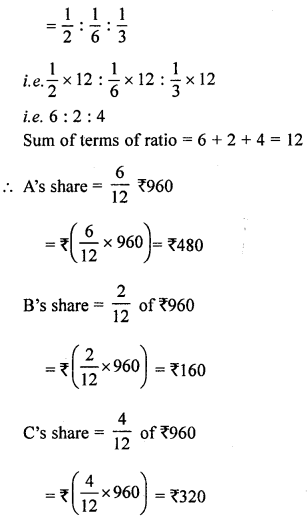Question 10.
A, B and C togetlier can do a piece of work in 15 days, B alone can do it in 30 days and C alone can do it in 40 days. In how many days will A alone do the work ?
Solution:
(A + B + C) can do a piece of work in = 15 days
B alone can do a piece of work in = 30 days
C alone can do a piece of work in = 40 days
∴ (A + B + C)’s 1 day work = $$\frac{1}{15}$$
B’s 1 day work = $$\frac{1}{30}$$
C’s 1 day work = $$\frac{1}{40}$$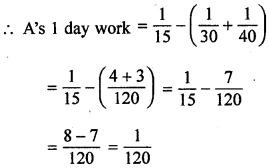Hence A can do a piece of work = 120 days.

Question 11.
A, B and C working together can plough a field in $$4 \frac{4}{5}$$ days. A and C together can do it in 8 days. How long would B working alone take to plough the field ?
Solution:
(A + B + C) can plough a field in = $$4 \frac{4}{5}$$ days = $$\frac{24}{5}$$ days
(A + C) can plough a field in = 8 days
∴ (A + B + C)’s 1 day work = $$\frac{5}{24}$$ days
(A + C)’s 1 day work = $$\frac{1}{8}$$ days
∴ B’s 1 day work = $$\frac{5}{24}-\frac{1}{8}$$ = $$\frac{5-3}{24}=\frac{2}{24}=\frac{1}{12}$$
Hence B can do the work in = 12 days.

Question 12.
A and B together can build a wall in 10 days; B and C working together can do it in 15 days; C and A together can do it in 12 days. How long will they take to finish the work, working altogether? Also find the number of days taken by each to do the same work, working alone.
Solution:
(A + B)’s can build a wall in = 10 day
(B + C)’s can build a wall in = 15 days
(C + A)’s can build a wall in = 12 days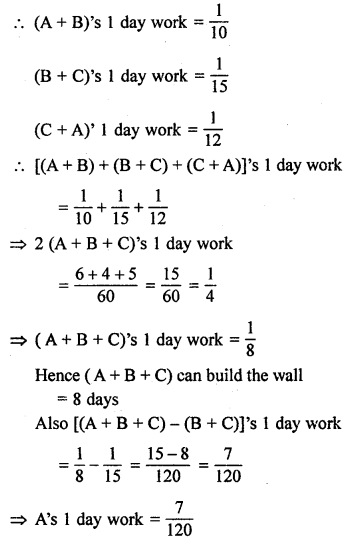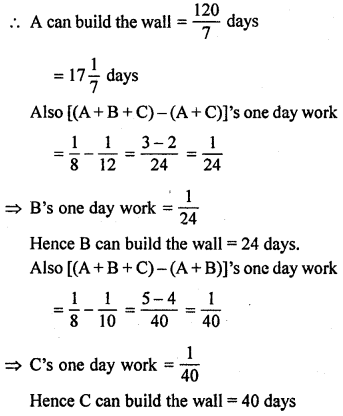Question 13.
A pipe can fill a tank in 12 hours. By mistake, a waste pipe in the bottom is left opened and the tank is filled in 16 hours. If the tank is full, how much time will the waste pipe take to empty it?
Solution:
Pipe can fill a tank in = 12 hours
By mistake pipe can fill a tank in = 16 hours
∴ In 1 hour fill a tank = $$\frac{1}{12}$$ part
By mistake In 1 hour fill a tank = $$\frac{1}{16}$$ part
portion of the tank emptied by the waste pipe in one hour = $$\frac{1}{12}-\frac{1}{16}=\frac{3-2}{48}=\frac{1}{48}$$
Hence for the tank is full, time will the waste pipe take to empty = 48 hours.